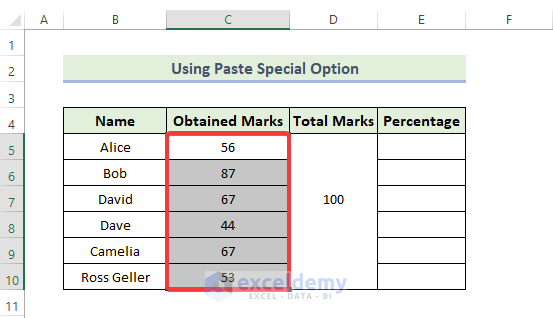# How to Divide a Value to Get a Percentage in Excel (5 Suitable Examples)

Get FREE Advanced Excel Exercises with Solutions!

If you are looking for some special tricks to divide in Excel to get a percentage, you’ve come to the right place. In Microsoft Excel, there are numerous ways to divide to get a percentage. In this article, we’ll discuss five ways to divide in Excel to get a percentage. Let’s follow the complete guide to learn all of this.

## How to Divide a Value to Get a Percentage in Excel: 5 Suitable Examples

We will use five effective and tricky ways to divide in Excel to get a percentage in the following section. This section provides extensive details in five ways. You should learn and apply all of these, as they improve your thinking capability and Excel knowledge.

### 1. Divide by Copying a Formula to Get a Percentage in Excel

It is the quickest and simplest way to divide in Excel to get a percentage. Here, we have a dataset containing different student marks. We must follow the following process if we wish to calculate each student’s percentage of marks.📌 Steps:

• Firstly, we will use the following formula in the cell F5:

`=(C5+D5)/E5`

Here, cells C5, and D5 show the marks of each student. The Total Marks are shown in cell E5.

• Press Enter.• Next, go to the Home tab, and select the Percentage symbol under the Number group.• Consequently, we can convert the data into percentage terms.• After that, drag down or double-click the Fill Handle icon to use Excel’s AutoFill feature.
• Finally, we will get the percentage values in the corresponding cells.### 2. Divide Using Array Formula in Excel

Here, we use another effective way to divide in Excel to get a percentage using array formulas. You have to follow the following steps to do this.

📌 Steps:

• Firstly, we will use the following formula in the cell F5:

`=(C5:C10+D5:D10)/E5:E10`

Here, the range of cells C5:C10, and D5:D10 show the marks of each student. The total marks are shown in the range of cells E5:E10.

• Press Enter.• Next, go to the Home tab, and select the Percentage symbol under the Number group.• Finally, we will get the percentage values in the corresponding cells.### 3. Divide Using a Constant Number to Get a Percentage

Here, we use another effective way to divide in Excel to get a percentage. We are going to divide the column’s values by a constant number. You have to follow the following steps to do this.

📌 Steps:

• Firstly, we will use the following formula in the cell F5:

`=(C5+D5)/200`

Here, cells C5, and D5 show the marks of each student. The Total mark is 200.

• Press Enter.• Next, go to the Home tab, and select the Percentage symbol under the Number group.• Consequently, we can convert the data into percentage terms.• After that, drag down or double-click the Fill Handle to use Excel’s AutoFill feature.
• Finally, we will get the percentage values in the corresponding cells.### 4. Using Absolute Cell Reference in Excel

Now, we use another quickest way to divide in Excel to get a percentage. We are going to divide the column’s values by a constant number using cell absolute reference to get a percentage. You have to follow the following steps to do this.

📌 Steps:

• Firstly, we will use the following formula in the cell F5:

`=(C5+D5)/\$E\$7`

Here, cells C5, and D5 show the marks of each student. The Total marks are shown in cell E7.

• Press Enter.• Next, go to the Home tab, and select the Percentage symbol under the Number group.• Consequently, we can convert the data into percentage terms.• After that, drag down or double-click the Fill Handle icon to use Excel’s AutoFill feature.
• Finally, we will get the percentage values in the corresponding cells.### 5. Divide by Utilizing Paste Special Command to Get a Percentage

Now, we use another quickest and most effective way to divide in Excel to get a percentage. We are going to divide the column values by a constant number using the paste special option to get a percentage. You have to follow the following steps to do this.

📌 Steps:

• Firstly, select the range of the data C5:C10 and press ‘Ctrl+C’.

###• Then, on the percentage column press Ctrl+V’ or you have to right-click and select the appropriate paste type like the following.• The percentage column will look like this after pasting the data.• Copy the cell D7 by pressing Ctrl+C’.• Next, select the range of the data C5:C10 and you have to right-click. Then click on the Paste Special option.• When the Paste Special dialog box appears, click on the Divide option under the Operation. Click on OK.• As a consequence, you will get the following output.• Next, go to the Home tab, and select the Percentage symbol under the Number group.• Finally, we will get the percentage values like the following.## Conclusion

That’s the end of today’s session. I strongly believe that from now you may be able to divide in Excel to get a percentage. If you have any queries or recommendations, please share them in the comments section below.

## What is ExcelDemy?

ExcelDemy Learn Excel & Excel Solutions Center provides free Excel tutorials, free support , online Excel training and Excel consultancy services for Excel professionals and businesses. Feel free to contact us with your Excel problems.Welcome to my Profile. I am working on and researching Microsoft Excel right now, and I will be posting articles about it here. I received a B.Sc. in Naval Architecture and Marine Engineering from the Bangladesh University of Engineering and Technology (BUET). Having studied naval architecture, I have a strong interest in research and development. Always try to learn from different sources and come up with creative solutions.

We will be happy to hear your thoughtsAdvanced Excel Exercises with Solutions PDF